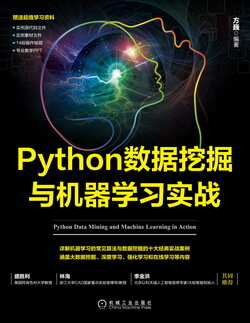# Python 数据挖掘与机器学习实战 (60)：回归分析介 3.4(用 Python 实现多元线性回归)

$Sales={\beta }_{0}+{\beta }_{1}TV+{\beta }_{2}Radio+{\beta }_{3}Newspaper\phantom{\rule{1em}{0ex}}\phantom{\rule{1em}{0ex}}\phantom{\rule{1em}{0ex}}\phantom{\rule{1em}{0ex}}\phantom{\rule{1em}{0ex}}\phantom{\rule{1em}{0ex}}\phantom{\rule{1em}{0ex}}\left(3-7\right)$$Sales=\beta_0+\beta_1TV+\beta_2Radio+\beta_3Newspaper\quad\quad\quad\quad\quad\quad\quad(3-7)$